# Thermal Imaging-based Physiological and Affective computing (TIPA)¶

## TIPA Opensource project¶

Created: August 2019 (very initial stage)
Author(s): Dr. Youngjun Cho (Assistant Professor, Department of Computer Science, University College London, UCL)

This project is to support the ACII 2019's tutorial on Thermal Imaging-based Physiological and Affective computing

Full source code: https://github.com/deepneuroscience/TIPA
Temporary TIPA opensource project website: http://youngjuncho.com/TIPA

## Key Reference¶

 Youngjun Cho and Nadia Bianchi-Berthouze. 2019. Physiological and Affective Computing through Thermal Imaging: A Survey. arXiv:1908.10307 [cs], http://arxiv.org/abs/1908.10307

### Further Technical References¶

 Cho, Y., Julier, S.J., Marquardt, N. and Bianchi-Berthouze, N., 2017. Robust tracking of respiratory rate in high-dynamic range scenes using mobile thermal imaging. Biomedical optics express, 8(10), pp.4480-4503. https://doi.org/10.1364/BOE.8.004480

 Cho, Y., Julier, S.J. and Bianchi-Berthouze, N., 2019. Instant Stress: Detection of Perceived Mental Stress Through Smartphone Photoplethysmography and Thermal Imaging. JMIR mental health, 6(4), p.e10140. https://doi.org/10.2196/10140

 Cho, Y., Bianchi-Berthouze, N. and Julier, S.J., 2017. DeepBreath: Deep learning of breathing patterns for automatic stress recognition using low-cost thermal imaging in unconstrained settings. In 2017 Seventh International Conference on Affective Computing and Intelligent Interaction (ACII) (pp. 456-463). IEEE. https://doi.org/10.1109/ACII.2017.8273639

 Cho, Y., Bianchi-Berthouze, N., Marquardt, N. and Julier, S.J., 2018. Deep Thermal Imaging: Proximate Material Type Recognition in the Wild through Deep Learning of Spatial Surface Temperature Patterns. In Proceedings of the 2018 CHI Conference on Human Factors in Computing Systems, ACM. https://doi.org/10.1145/3173574.3173576

Let's start!

## Basic tutorial¶

### 1. Import TIPA libraries¶

In :
import sys
from platform import python_version
# sys.path.insert(0,'./TIPA_library/')

from TIPA_library.main.data_preparation import *
from TIPA_library.main.thermal_image_processing import *
from TIPA_library.utils import timshow as tim
from TIPA_library.utils import rvs


The TIPA project mainly uses the TIPA frame protocol below by default.

For FLIR ONE (SDK) users, you can simply implement the code from the link below. https://github.com/deepneuroscience/DeepThermalImaging/tree/master/example%20code%20for%20FLIR%20One%20sdk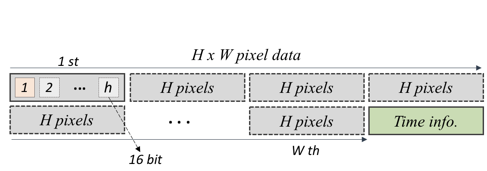Figure 1. TIPA (Thermal Imaging-based Physiological and Affective computing) Project Dataframe protocol

#### Example Dataset¶

We provide example data.

Unzip, move them to a directory (./data)
./data/example_data.dat
./data/example_data_in_front_of_building.dat

In :
# The matrix size has to be known in advance. e.g.320 x 240
# data = data_preparation_TIPA_protocol('./data/example_data.dat',320,240)
data = data_preparation_TIPA_protocol('./data/example_data_in_front_of_building.dat',320,240)
# print(data.time_stamp)


For the ones using other thermal cameras,
(you may need to convert the ascii format, e.g. *.asc to a matrix.)
contact youngjun[dot]cho[at]ucl.ac.uk for any quiries

In :
# data = data_preparation_raw_matrix(matrix, framerate)


### 3. Manual Inspection of the loaded thermal matrices¶

#### Tool 1: interactive_thermal_matrix_view

simply, you can examine temperatures on your thermal image with your cursor.

In :
from ipywidgets import interact, interactive, fixed, interact_manual
import ipywidgets as widgets
import matplotlib.pyplot as plt

%matplotlib notebook
def update(fig):
fig.canvas.draw()

def interactive_thermal_matrix_view(matrix):
fig= plt.figure()
plt.imshow(matrix)

interact(update(fig))

frame_number = 1
interactive_thermal_matrix_view(data.thermal_matrix[:,:,frame_number])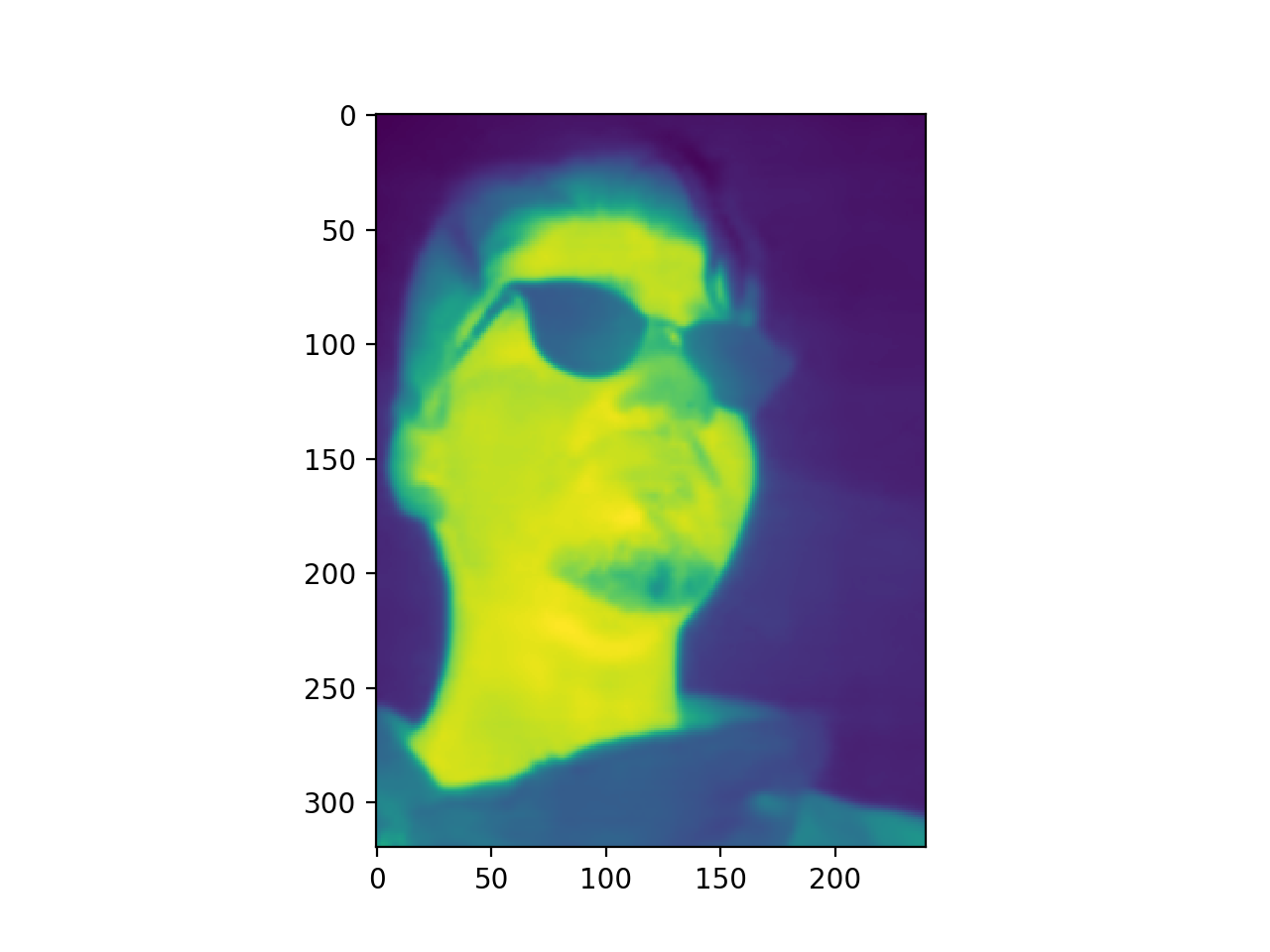#### Tool 2: Interactive ImShow Cond1 for multiple frames

simply, you can change the value on the slider (frame_num). e.g. frame number = 100

In :
from ipywidgets import interactive, FloatRangeSlider, Output, VBox
%matplotlib inline

interactive_plot = interactive(data.interactive_imshow_cond,  frame_number=(0, data.thermal_matrix.shape-1))
output = interactive_plot.children[-1]
output.layout.height = '320px'
interactive_plot
# VBox([range_slider,interactive_plot])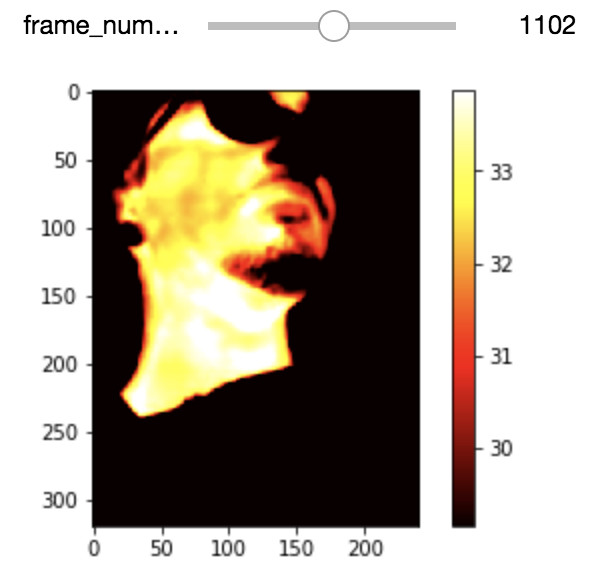#### Tool 3: Interactive ImShow Cond2 for multiple frames with a function to select your thermal range of interest

in short, you can change the value on the slider (frame_num). e.g. frame number = 100
and you can adjust the thermal (temperature) range of interest

In :
from ipywidgets import interactive, FloatRangeSlider, Output, VBox, Layout
%matplotlib inline

# layout = Layout(width='500px')
range_slider = widgets.FloatRangeSlider(
value=[0, +50],
min=0., max=+60., step=0.1,
description='thermal range of interest',
#     layout=layout
)
# range_slider

interactive_plot = interactive(data.interactive_imshow_cond2,  frame_number=(0, data.thermal_matrix.shape-1), thermal_range=range_slider)
output = interactive_plot.children[-1]
output.layout.height = '320px'
interactive_plot
# VBox([range_slider,interactive_plot])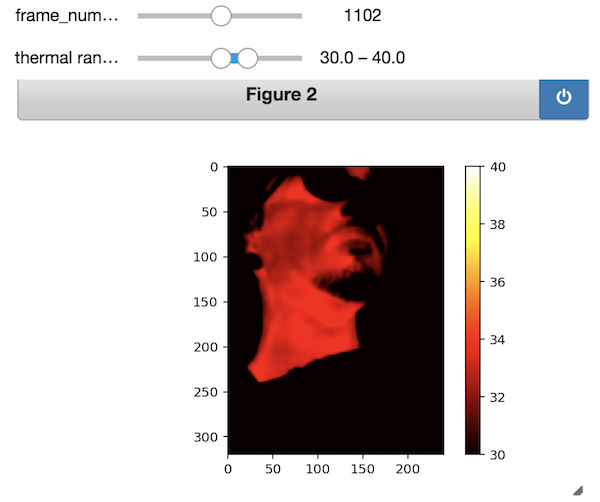#### Tool 4: Interactive ImShow Cond 3¶

##### for multiple frames with the optimal quantization to automatically select your thermal range of interest

in short, you can change the value on the slider (frame_num). e.g. frame number = 100
and this will automatically select your thermal (temperature) range of interest
We will come back here again on the preprocessing section

###### [Key reference]

Cho, Y., Julier, S.J., Marquardt, N. and Bianchi-Berthouze, N., 2017. Robust tracking of respiratory rate in high-dynamic range scenes using mobile thermal imaging. Biomedical optics express, 8(10), pp.4480-4503. https://doi.org/10.1364/BOE.8.004480

In :
from ipywidgets import interactive, FloatRangeSlider, Output, VBox, Layout
%matplotlib inline

interactive_plot = interactive(data.interactive_imshow_cond3,  frame_number=(0, data.thermal_matrix.shape-1))
output = interactive_plot.children[-1]
output.layout.height = '320px'
interactive_plot
# VBox([range_slider,interactive_plot])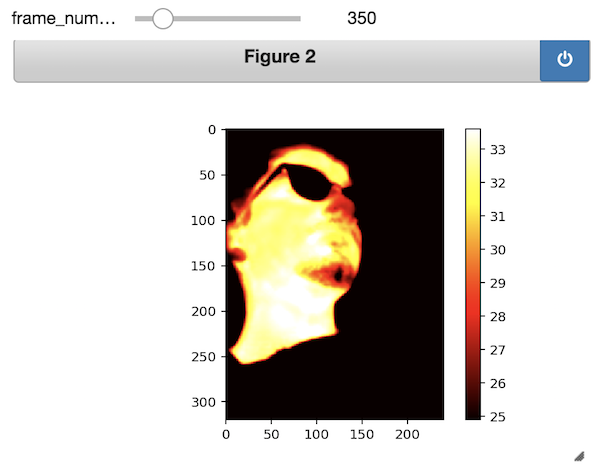### 4. Computational Pipeline¶

Here, we focus on the case where an automatic ROI tracking method is used. For the ROI selection, a ROI can be chosen either manually or automatically. (here we select it manually)